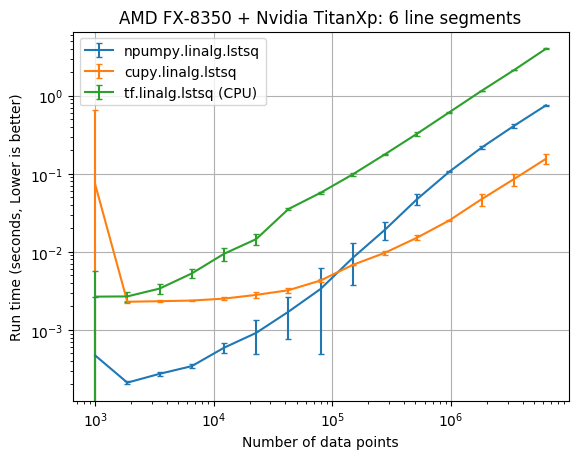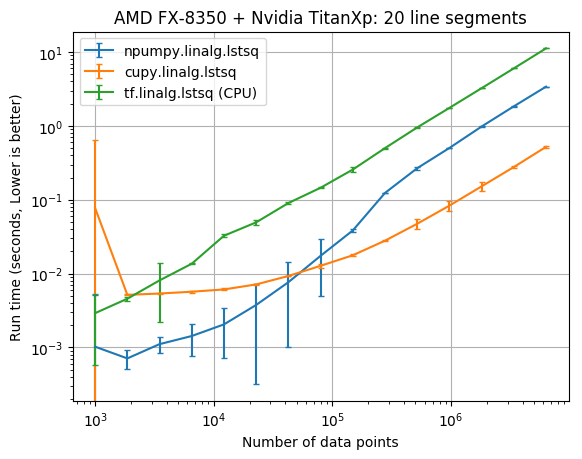# Compare lstsq performance in Python

## April 28, 2019

Edit 2019-05-09: The benchmark has been updated to include the latest CuPy syntax for cupy.linalg.lstsq.

CuPy is a GPU accelerated version of NumPy that is very easy to use. I just submited a PR which adds cupy.linalg.lstsq, and naturally I wanted to compare the least squares performance to numpy.linalg.lstsq. I also compared tensorflow.linalg.lstsq as a reasonable alternative. Hopefully this will provide some insight into when it will pay off to solve least squares problems with CuPy instead of NumPy.

I only have one computer that I was able to run this on so far. I’ll update the post if I can run this benchmark on different hardware.

# Least squares solvers

NumPy and CuPy use singular value decomposition (SVD) to solve least squares problems. NumPy defaults to the gelsd lapack routine which is a divide-and-conquer SVD strategy. TensorFlow’s lstsq defaults to using a Cholesky decomposition which should be faster than SVD.

# Benchmark

I have a small benchmark problem which performs piecewise linear least squares fits to a sine wave. The benchmark performs fits with known breakpoint locations for 6 and 20 line segments (which translates to solving least squares problem for 6 or 20 unknown parameters). The number of data points was varied from 100 to 6,309,573. This is an odd number, because I couldn’t solve 10**7 data points in the 12 Gb of my GPU’s memory. The code to run the benchmark is available here, and the process to run the code was

python3 sine_benchmark_fixed_six_break_points.py
python3 sine_benchmark_fixed_six_break_points_TFnoGPU.py
python3 sine_benchmark_fixed_twenty_break_points.py
python3 sine_benchmark_fixed_twenty_break_points_TFnoGPU.py
python3 plot_results.py


This code basically runs the following:

import tensorflow as tf
import cupy as cp
import numpy as np
import pwlf
from time import time
import os

n_data = 10**6  # number of data points
np.random.seed(256)
# generate sin wave data
x = np.linspace(0, 10, num=n_data)
y = np.sin(x * np.pi / 2)
# add noise to the data
y = np.random.normal(0, 0.05, size=n_data) + y
my_pwlf = pwlf.PiecewiseLinFit(x, y)
A = my_pwlf.assemble_regression_matrix(breaks, my_pwlf.x_data)
Acp = cp.asarray(A)
ycp = cp.asarray(y)

# numpy.linalg.lstsq
t0 = time()
beta_np, _, _, _ = np.linalg.lstsq(A, y, rcond=1e-15)
t1 = time()

# cupy.linalg.lstsq
t2 = time()
beta_cp = cp.linalg.lstsq(Acp, ycp)
t3 = time()
Atf = tf.convert_to_tensor(A)
ytf = tf.convert_to_tensor(y.reshape(-1, 1))
beta_tf_fast = tf.linalg.lstsq(Atf, ytf, fast=True)
beta_tf_not_fast = tf.linalg.lstsq(Atf, ytf, fast=False)

with tf.Session():
# tf.linalg.lstsq fast=True
t4 = time()
beta_tf = beta_tf_fast.eval()
t5 = time()


I’ve ran the benchmark on the following computers:

CPU GPU OS TF & NumPy built from source
AMD FX-8350 NVIDIA TITAN Xp Linux Yes

# Results

The results below compare the run time of the fits against the number of data points. The vertical error bars denote the 10th and 90th percentile, considering a Normal distribution with 90% confidence (from the 10 replicate runs).CPU/GPU lstsq parameters Data points CuPy x faster NumPy
AMD FX-8350/NVIDIA TITAN Xp 6 6,309,573 4.84
AMD FX-8350/NVIDIA TITAN Xp 20 6,309,573 6.54

# Disucssion

It appears that the CuPy least squares solver wasn’t faster than NumPy until there was at least 100,000 data points. With 6,309,573 data points cupy.linalg.lstsq was about 6 times faster than numpy.linalg.lstsq.

I should really run this benchmark on a computer with Intel CPU + NVIDIA GPU. Especially since a previous post hinted that TensorFlow performs significantly better on an Intel CPU, and a Cholesky decomposition should be faster than SVD.

NumPy was built from source using Intel MKL on the AMD FX-8350 which isn’t the fastest, however it is the best supported…

# Acknowledgements

The TITAN Xp used for this work was donated by the NVIDIA Corporation.